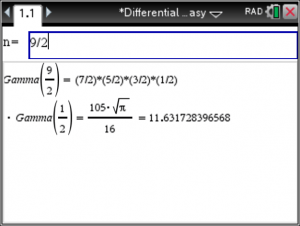# Gamma and Beta Function – Step by Step – for the TiNSpire CX

To compute the Gamma Function – Step by Step – using the TiNspire CX start the Differential Equation Made Easy app at https://www.tinspireapps.com/?a=DEQME  , go to menu option EXTRAS and select GAMMA FUNCTION. You will be prompted to enter value for n as shown below.

Entering in example n=9 yields 8! or 40320 as the Gamma Value.You may also enter .5 – value such as 4.5 or 9/2 into the Gamma Function, see below.The Beta Function can easily be computed using the Gamma Function upon entering two values x and y for the Beta Function. Just select BETA FUNCTION under the EXTRAS menu.

Below we are entering x=5 and y = 4 to get the correct Beta Function value of 1/280 :As you can see the Gamma and Beta Functions can be computed easily using the Differential Equations Made Easy. Values are computed step and step and are always correct. Even for large values of x and y and n .

Posted on Categories differential equation